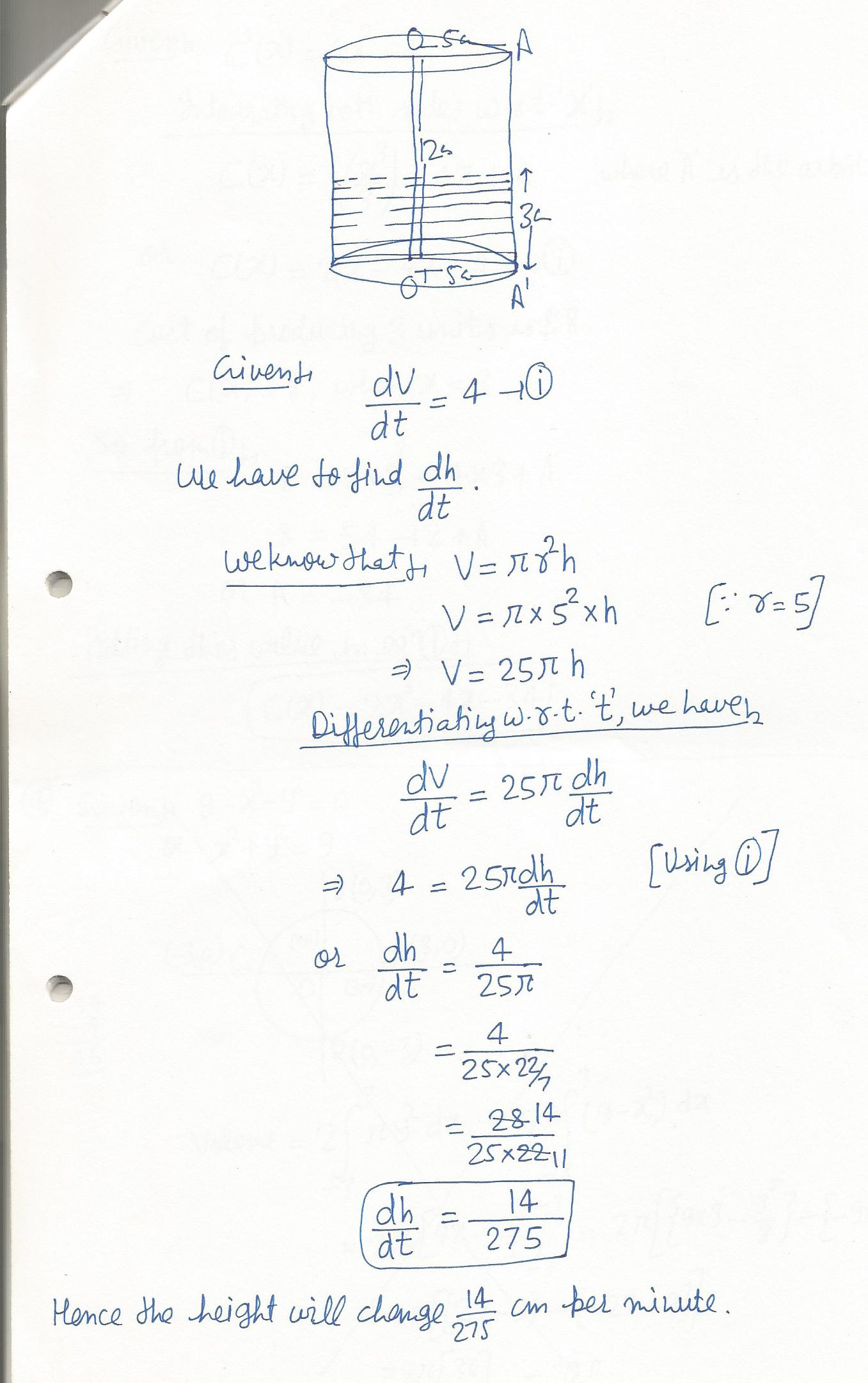8051

Mathematics Calculus Level: Misc Level

A cylindrical can of radius 5cm and height 12 cm is leaking water at a rate of 4 cm 3 (cubed) per minute. How fast is the height of the water changing when the water level is 3 cm from the bottom of the can.(this is a,,related rates,, problem. Volume of a cylinder is given by V=pie r2(squared)h.)